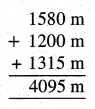# PSEB 5th Class Maths MCQ Chapter 6 Measurement

Punjab State Board PSEB 5th Class Maths Book Solutions Chapter 6 Measurement MCQ Questions and Answers.

## PSEB 5th Class Maths Chapter 6 Measurement MCQ Questions

Multiple Choice Questions

Tick (✓) the right answer :

Question 1.
Convert 8 m into centimetres,
(a) 80 cm
(b) 800 cm
(c) 8000 cm
(d) 8 cm.
ans:
(b) 800 cm

Question 2.
Convert 16 kl into litres.
(a) 160 l
(b) 1600 l
(c) 16000 l
(d) 160000 l
Ans:
(c) 16000 lQuestion 3.
Convert 10 da.g.into grams.
(a) 100 g
(b) 1000 g
(c) 10 g
(d) 10000 g.
Ans:
(c) 10 g

Question 4.
How many kg are there in 1000 g?
(a) 100 kg
(b) 10 kg
(c) 20 kg
(d) 1 kg.
Ans:
(d) 1 kg.

Question 5.
Decimal formation of 3 l 175 ml.
(a) 31.75 l
(b) 317.5 l
(c) 3.175 l
(d) 0.3175 l
ans:
(c) 3.175 lQuestion 6.
3.5 km = …………… m
(a) 350 m
(b) 3500 m
(c) 35 m
(d) 0.350 m.
Ans:
(b) 3500 m

Question 7.
Which unit is used by a shopkeeper to weigh vegetables ?
(a) litre and kl
(b) metre and km
(c) gram and kg
(d) none.
Ans:
(c) gram and kg

Question 8.
Which unit is used to measure liquids ?
(a) litre
(b) kilometre
(c) metre
(d) none.
Ans:
(a) litreQuestion 9.
Kanwal bought 6 kg potatoes, 3 kg 500 g onions and 500 g tomatoes from the market How many kg of vegetables had he bought ?
(a) 10 kg
(b) 6 kg
(c) 3 kg
(d) 11 kg.
ans:
(a) 10 kg

Question 10.
Harpreet has bought 10 m cloth, he uses 6 m 50 cm cloth for her suit. How much cloth is left ?
(a) 2 m 50 cm
(b) 4 m
(c) 4 m 50 cm
(d) 3 m 50 cm.
ans:
(d) 3 m 50 cm

Question 11.
How many metre are in one milimetre ?
(a) $$\frac{1}{100}$$
(b) $$\frac{1}{1000}$$
(c) $$\frac{1}{10}$$
(d) 100.
Ans:
(b) $$\frac{1}{1000}$$Question 12.
How many centimetres are in one hectometres ?
(a) 1000
(b) 10,000
(c) 100
(d) $$\frac{1}{1000}$$
Ans:
(b) 10,000

Question 13.
How many hectogram are in one kilogram ?
(a) 100
(b) $$\frac{1}{100}$$
(c) 10
(d) $$\frac{1}{10}$$
Ans.
(c) 10

Question 14.
How many decalitres are in one kilolitre ?
(a) 1000
(b) 500
(c) 200
(d) 100
Ans.
(d) 100Question 15.
How many mililitres are in one decilitre ?
(a) 10
(b) 100000
(c) 100
(d) 1000
Ans:
(c) 100

Question 16.
How many days are in a leap year ?
(a) 364
(b) 366
(c) 365
(d) 363
Ans:
(b) 366

Question 17.
How many days are there in the month of February in a leap year ?
(a) 28
(b) 30
(c) 29
(d) 31.
Ans:
(c) 29Question 18.
Write 3:10 pm according to 24 hour clock ?
(a) 23:10
(b) 25:10
(c) 15:10
(d) 13:10.
Ans:
(c) 15:10

Question 19.
Write 22:25 according to 12 hour clock.
(a) 10:25 PM
(b) 12:25 AM
(c) 12:25 PM
(d) 9: 25 PM.
Ans:
(a) 10:25 PM

Question 20.
How many seconds make one hour ?
(a) 60
(b) 3600
(c) 360
(d) 300.
Ans:
(b) 3600

Question 21.
The distance of your village dispensary from your school is 2 km, village community hall 955 m and distance of guruduara is 1500 m. Which of these is at a maximum distance from your school ?
(a) Dispensary
(b) Community centre
(c) Guruduara
(d) All are at equal distances.
Ans:
(a) DispensaryQuestion 22.
See Carefully and tell :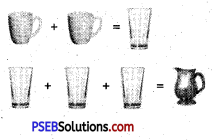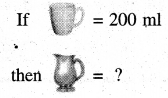(a) less than 500 ml
(b) In-between 500 ml and 1 litre
(c) In between 1 l and 2 l
(d) More than 2 l
Ans:
(c) In between 1 l and 2 l

Question 23.
How many metres are there in 3.5 kilometres ?
Ans:
3.5 kilometres = 3.5 × 1000 metre
= 3500 metre.

Question 24.
How many seconds are there in a day ?
Ans:
1 day = 24 hours = 24 × 60 minutes
= 24 × 60 × 60 seconds = 86400 seconds.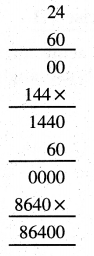Question 25.
The map of a village which is at a distance from a city is given. Simran is moving in the village on his cycle.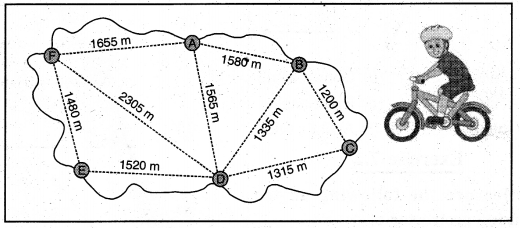Find the distance covered by Simran :
(a) From D to A (passing through B)
(b) From A to D (passing through B and C)
Ans:
(a) Distance covered by Simran from D to A (passing through B) = DB + BA.
= 1335 m + 1580 m
= 2915 m

(b) Distance covered by Simran from A to D (passing through B and C)
= AB + BC + CD
= 1580m + 1200m + 1315 m
= 4095m